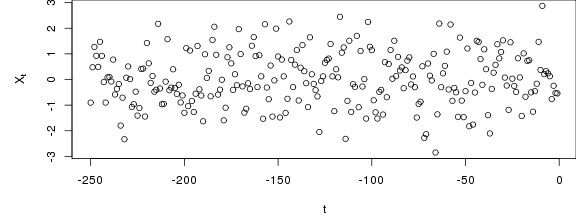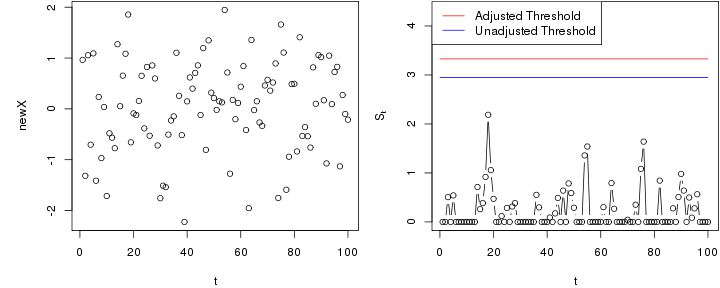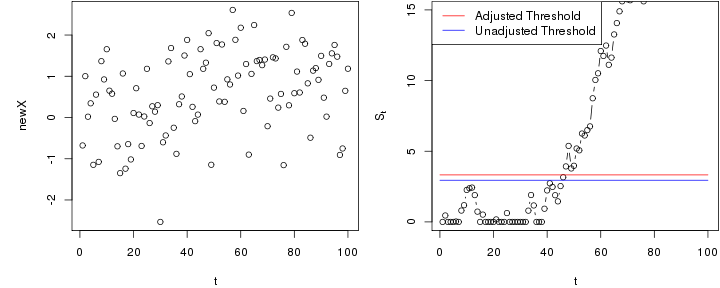# CUSUM chart with estimated in-control state

## Using normality assumptions

The following is an example of an application to a basic CUSUM chart, assuming that all observations are normally distributed.

Based on $$n$$ past in-control observations $$X_{-n},\dots,X_{-1}$$, the in-control mean can be estimated by $$\hat \mu = \frac{1}{n}\sum_{i=-n}^{-1} X_i$$ and the in-control variance by $$\hat \sigma^2=\frac{1}{n-1}\sum_{i=-n}^{-1} (X_i-\hat \mu)^2$$. For new observations $$X_1,X_2,\dots$$, a CUSUM chart based on these estimated parameters is then defined by $S_0=0, \quad S_t=\max\left(0,\frac{S_{t-1}+X_t-\hat \mu-\Delta/2}{\hat \sigma}\right).$

The following generates a data set of past observations (replace this with your observed past data).

X <-  rnorm(250)Next, we initialise the chart and compute the estimates needed for running the chart - in this case $$\hat \mu$$ and $$\hat \sigma$$.

library(spcadjust)
chart <- new("SPCCUSUM",model=SPCModelNormal(Delta=1));
xihat <- xiofdata(chart,X)
str(xihat)

## List of 3
##  $mu: num 0.0251 ##$ sd: num 1.05
##  \$ m : int 250


## Calibrating the chart to a given average run length (ARL)

We now compute a threshold that with roughly 90% probability results in an average run length of at least 100 in control. This is based on parametric resampling assuming normality of the observations.

cal <- SPCproperty(data=X,nrep=50,
property="calARL",chart=chart,params=list(target=100),quiet=TRUE)
cal

## 90 % CI: A threshold of 3.328 gives an in-control ARL of at least
##   100.
## Unadjusted result:  2.949
## Based on  50 bootstrap repetitions.


The number of bootstrap replications (the argument nrep) shoud be increased for real applications. Use the parallell option to speed up the bootstrap by parallel processing.

## Run the chart

Next, we run the chart with new observations that are in-control.

newX <- rnorm(100)
S <- runchart(chart, newdata=newX,xi=xihat)


Then we plot the data and the chart.

par(mfrow=c(1,2),mar=c(4,5,0.1,0.1))
plot(newX,xlab="t")
plot(S,ylab=expression(S[t]),xlab="t",type="b",ylim=range(S,cal@res+1,cal@raw))
lines(c(0,100),rep(cal@res,2),col="red")
lines(c(0,100),rep(cal@raw,2),col="blue")newX <- rnorm(100,mean=c(rep(0,50),rep(1,50)))